# Yosida representation theorem

Letbe a topological space,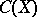the set of continuous real-valued functions on(cf. Continuous functions, space of). Using the pointwise defined partial order: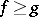if and only if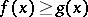for all,becomes a Riesz space. The question arises whether it is possible to represent an arbitrary Riesz space by continuous functions with this order relation where, possibly, more general (extended) functions that can also take the values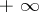andmay be used. Answers are given by various representation theorems. Below the Yosida representation theorem for the case of Archimedean Riesz spaces with a strong unit is described. For the Yosida representation theorem for Riesz spaces, whereis a weak unit, see [a1], and for the more general Johnson–Kist representation theorem, see [a2].

A strong unit in a Riesz spaceis an element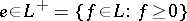such that for allthere is an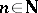such that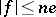, i.e. the principal ideal generated byshould equal the whole space. A weak unit inis an element ofsuch that the principal band generated byis all of.

## The Riesz space.

Letbe a compact Hausdorff space. Thenis an Archimedean Riesz space and the function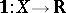,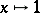for all, is a strong unit. Letbe a second compact Hausdorff space. The Banach–Stone theorem says that ifand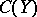are isomorphic as Riesz spaces, thenandare homeomorphic. As immediate corollaries one obtains that ifandare isomorphic as algebras (pointwise multiplication), thenandare homeomorphic; also, ifandare isomorphic as Banach spaces (sup-norm), thenandare homeomorphic.

A topological spaceis extremely disconnected if every open subsethas an open closure (i.e.is both open and closed). Nakano's theorem says thatis Dedekind complete if and only ifis extremely disconnected. It was also obtained independently by T. Ogasawara and M.H. Stone, cf. [a2].

## Representation of Archimedean Riesz spaces with strong unit.

Letbe an Archimedean Riesz space. Let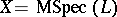be the set of maximal ideals of. For each, let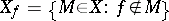. Define a topology onby taking theas a subbase (cf. Pre-base). The closed sets ofare the sets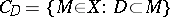, whereruns through all subsets of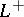. This topology is called the hull-kernel topology on. The construction is a fairly familiar one and occurs in several parts of mathematics. It is originally due to M.H. Stone. Depending on which sets of ideals are used, the mathematical specialism involved, and the ideosyncracies of authors it is also called the Zariski topology, Gel'fand topology, Gel'fand–Kolmogorov topology, Jacobson topology, Grothendieck topology, etc.

From now on, lethave a strong unit. For each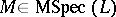,and there is a unique homomorphism of Riesz spacessuch that. Using this, one defines for every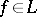a function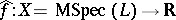by. The number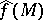can also be described as the unique real number such that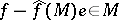. One now has the following representation theorem (K. Yosida, S. Kakutani, M.G. Krein, S.G. Krein, H. Nakano). Let,,be as just described. Then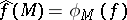defines a continuous function onand the mapping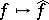is a Riesz isomorphism ofonto a Riesz subspace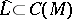. There are a number of complementary facts. Using the Stone–Weierstrass theorem one obtains that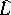is norm dense in; it is then also order dense.

Given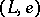, whereis a weak unit,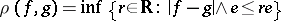defines a metric on, called the uniform metric. Ifis complete with respect to this metric,is called uniformly closed. A further addition to the representation theorem is then thatis isomorphic to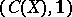if and only ifis a strong unit andis uniformly closed. This last statement, together with that fact thatis isomorphic to a sub-Riesz space of(ifis a strong unit), is also referred to as the Krein–Kakutani theorem.

A final complement to the Yosida representation theorem is that ifhas the principal projection property, i.e.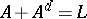for every principal band, thenis zero dimensional andcontains all locally constant functions on. This can also be called the Freudenthal spectral theorem, [a3], in the sense that that theorem in its traditional formulation is an immediate consequence of this result, cf. (the editorial comments to) Riesz space.